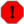## f Statement (or Function Table Statement)

f Statement (or Function Table Statement) — Causes a GEN subroutine to place values in a stored function table.

## Description

This causes a GEN subroutine to place values in a stored function table for use by instruments.

## Syntax

`f p1  p2  p3  p4  p5 ... PMAX`

## Performance

p1 -- Table number by which the stored function will be known. A negative number requests that the table be destroyed.

p2 -- Action time of function generation (or destruction) in beats.

p3 -- Size of function table (i.e. number of points) Must be a power of 2, or a power-of-2 plus 1 if this number is positive. Maximum table size is 16777216 (224) points.

p4 -- Number of the GEN routine to be called (see GEN ROUTINES). A negative value will cause rescaling to be omitted.

p5 ... PMAX -- Parameters whose meaning is determined by the particular GEN routine.

### Special Considerations

Function tables are arrays of floating-point values. You can create a simple sine wave using the line:

f 1 0 1024 10 1

This table uses GEN10 to fill the table.

Historically, due to older platform constraints, Csound could only accept tables whose size was a power of two. This limitation has been removed in recent versions, and you can freely create tables of any size. However, to create a table whose size is not a power of two (or power of two plus one), you must specify the size as a negative number.

NoteNot all opcodes accept tables whose size is not a power of two, since they may depend on this for internal optimization.

For arrays whose length is a power of 2, space allocation always provides for 2n points plus an additional guard point. The guard point value, used during interpolated lookup, can be automatically set to reflect the table's purpose: If size is an exact power of 2, the guard point will be a copy of the first point; this is appropriate for interpolated wrap-around lookup as in oscili, etc., and should even be used for non-interpolating oscil for safe consistency. If size is set to 2 n + 1, the guard point value automatically extends the contour of table values; this is appropriate for single-scan functions such in envplx, oscil1, oscil1i, etc.

The size of the table is used as a code to tell Csound how to fill this guard-point. If the size is exactly power-of-two, then the guard point contains a copy of the first point on the table. If the size is power-of-two plus one, Csound will extend the contour of the function stored in the table for one extra point.

Table space is allocated in primary memory, along with instrument data space. The maximum table number used to be 200. This has been changed to be limited by memory only. (Currently there is an internal soft limit of 300, this is automatically extended as required.)

An existing function table can be removed by an f statement containing a negative p1 and an appropriate action time. A function table can also be removed by the generation of another table with the same p1. Functions are not automatically erased at the end of a score section.

p2 action time is treated in the same way as in i statements with respect to sorting and modification by t statements. If an f statement and an i statement have the same p2, the sorter gives the f statement precedence so that the function table will be available during note initialization.

WarningThe maximum number of p-fields accepted in the score is determined by PMAX (a compilation time varible). PMAX is currently set to 1000. This may discard values entered when using GEN02. To overcome this, use GEN23 or GEN28 to read the values from a file.

An f 0 statement (zero p1, positive p2) may be used to create an action time with no associated action. Such time markers are useful for padding out a score section (see s statement) and for letting Csound run from realtime events only (e.g. using only MIDI input without score events). The time given is the number of seconds Csound will run. If you want Csound to run for 10 hours, use:

f0 36000

The simplest way to fill a table (f1) with 0's is:

f1 0 xx 2 0

where xx = table size.

The simplest way to fill a table (f1) with *any* single value is:

f1 0 xx -7 yy xx yy

where xx = table size and yy = any single value

In both of the above examples, table size (p3) must be a power of 2 or power-of-2 plus 1.